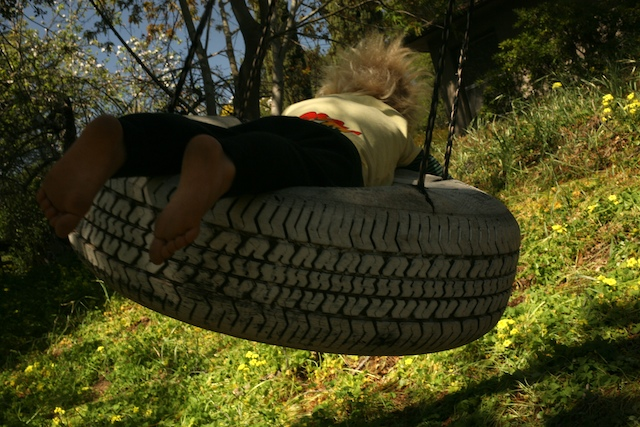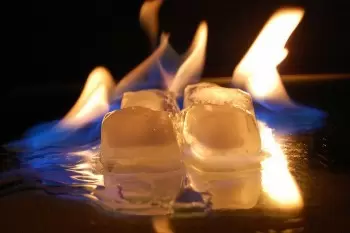Categories

## Work when volume or pressure is constant

There are a few common scenarios where we might want to calculate work in chemistry class, and it helps to be able to recognize them when they come up. We will discuss how work is calculated for these cases.

### Constant volume processes

Sometimes reactions or processes occur in a rigid, sealed container such as a bomb calorimeter. When there is no change in volume possible, it is also not possible for gases to do work because \Delta\text V =0ΔV=0delta, start text, V, end text, equals, 0. In these cases, \text {work}= 0work=0start text, w, o, r, k, end text, equals, 0 and change in energy for the system must occur in other ways such as heat.

### Bench (or stove) top reactions: Constant pressure processes

In chemistry, we will often be interested in changes in energy that occur during a chemical reaction at constant pressure. For example, you may run a reaction in an open beaker on the benchtop. These systems are at constant pressure because the pressure in the system can equilibrate with the atmospheric pressure of the surroundings.

Photograph of soup containing tomatoes, onions, and meat in a clear orange-ish broth. The metal pot is on a white stovetop, and the soup is being stirred with a black plastic spoon.Cooking soup in an open pot is another example of a chemical reaction at constant pressure!In this situation, the volume of the system can change during the reaction, so 0ΔV​= 0, does not equal, 0 and work is also non-zero. Heat can also be transferred between the system (our reaction) and the surroundings, so both work and heat must be considered when thinking about the energy change for the reaction.

The energy contribution from work becomes more significant when the reaction makes or consumes gases, especially if the number of moles of gas changes substantially between the product and the reactants.Other chemical processes result in only a small volume change, such as in the phase change from a liquid to a solid. In these cases, the energy change due to work will also be quite small, and may even be ignored when calculating the energy change. The relationship between work, heat, and other forms of energy transfer is further discussed in the context of the first law of thermodynamics.

Categories

## Example: Calculating work done on a gas

To illustrate how to use the equation for PV work, let’s imagine a bicycle pump. We will assume that the air in the bicycle pump can be approximated as an ideal gas in a piston. We can do work on the air in the pump by compressing it. Initially, the gas has a volume of 3.00L  We apply a constant external pressure of 1.10 atm to push down the handle of the bike pump until the gas is compressed to a volume of 2.50 L

How much work did we do on the gas?We can use the equation from the previous section to calculate how much work was done to compress the gas:

W = -Pexternal × ΔV

= -Pexternal ×(Vfinal × Vinitial)

If we plug in the values for Pexternal, Vfinal and Vinitial for our example, we get:

W = -1.10atm × (2.50L – 3.00L)

= -1.10atm × -0.05L

= 0.55L . atm

Let’s check the sign for the work to make sure it makes sense. We know the gas had work done on it, since the volume of the gas decreased. That means the value of work we calculated should be positive, which matches our result. Hooray! We can also convert our calculated work to Joules using the conversion factor:

w = 0.55 L.atm × 101.325 J / 1 L .atm = 56 J

Thus, we did 56 J f work to compress the gas in the bicycle pump from 3.00 L to 2.50 L

Categories

## Pressure-volume work: Work done by a gas

Gases can do work through expansion or compression against a constant external pressure. Work done by gases is also sometimes called pressure-volume or PV work for reasons that will hopefully become more clear in this section!Let’s consider gas contained in a piston. If the gas is heated, energy is added to the gas molecules. We can observe the increase in average kinetic energy of the molecules by measuring how the temperature of the gas increases. As the gas molecules move faster, they also collide with the piston more often. These increasingly frequent collisions transfer energy to the piston and move it against an external pressure, increasing the overall volume of the gas. In this example, the gas has done work on the surroundings, which includes the piston and the rest of the universe.To calculate how much work a gas has done (or has done to it) against a constant external pressure, we use a variation on the previous equation:

work = w = -Pexternal × ΔV

where Pexternal  is the external pressure (as opposed to the pressure of the gas in the system) and ΔV is the change in the volume of the gas, which can be calculated from the initial and final volume of the gas:

ΔV = Vfinal – Vinitial

Since work is energy, it has units of Joules(where 1 Joule = 1 kg.mm2 / s2)  You may also see other units used, such as atmospheres for pressure and liters for volume, resulting in L . atm, as the unit for work. We can convert L⋅atm, to convert to Joules using the conversion factor of 101.325J/ 1L.atm.

### The sign of work

As a matter of convention, negative work occurs when a system does work on the surroundings.

• When the gas does work the volume of a gas increases (\Delta \text V>0ΔV>0delta, start text, V, end text, is greater than, 0) and the work done is negative.
• When work is done on the gas, the volume of the gas decreases (\Delta \text V<0ΔV<0delta, start text, V, end text, is less than, 0) and work is positive.

One way to remember the sign convention is to always think about the change in energy from the point of view of the gas. When the gas expands against an external pressure, the gas has to transfer some energy to the surroundings. Thus, the negative work decreases the overall energy of the gas. When the gas is compressed, energy is transferred to the gas so the energy of the gas increases due to positive work.

Categories

## Pressure-volume work

The meaning of work in thermodynamics, and how to calculate work done by the compression or expansion of a gas.

## Key Points:

• Work is the energy required to move something against a force.
• The energy of a system can change due to work and other forms of energy transfer such as heat.
• Gases do expansion or compression work following the equation:work = −PΔV

## Introduction: Work and thermodynamics

When people talk about work in day-to-day conversation, they generally mean putting effort into something. You might “work on a school project” or “work to perfect your baseball pitch.” In thermodynamics, however, work has a very specific meaning: it is the energy it takes to move an object against a force. Work w, is one of the fundamental ways energy enters or leaves a system, and it has units of Joules (J).

When a system does work on the surroundings, the system’s internal energy decreases. When a system has work done on it, the internal energy of the system increases. Like heat, the energy change from work always occurs as part of a process: a system can do work, but doesn’t contain work.If the system is a child on a tire swing, we can do work on the system by giving it a push. We will be doing work against gravity, and this will increase the internal energy of the child on the swing,

To calculate work done by a constant force, we can use the following general equation:

work = force × displacementstart

For the purposes of chemistry class (as opposed to physics class), the most important takeaway from this equation is that work is proportional to the displacement as well as the magnitude of the force used. Different versions of the work equation can be used depending on the type of force involved. Some examples of doing work include:

• A person lifting books from the ground to a shelf does work against gravity.
• A battery pushing electrical current through a circuit does work against resistance.
• A child pushing a box along the floor does work against friction.

In thermodynamics, we are mainly interested in work done by expanding or compressing gases.

Categories

## What Does It Mean in Thermal Equilibrium?

When two systems are in thermal equilibrium, they have the same temperature. The extension of this principle fundamentally justifies using the thermometer and establishes the principles of its construction for its measurement.

The empirical definition is derived from the thermodynamics’ zeroth principle’s thermal equilibrium conditions in a more fundamental approach.

Categories

## How Is the Temperature Measured?

Different measurement systems depend on the application or whether very high or shallow values ​​are to be measured. However, the best known and used tool is the thermometer.

To determine the temperature of a system, it must be in thermodynamic equilibrium. Variations in a body’s thermal state cause changes in physical properties such as dilatation, the evolution of electrical resistance, the creation of electromotive forces, pressure changes, volume changes in gas, etc.

Consequently, variations in these properties allow them to be used for the construction of instruments that detect temperature variations.

Previously, the mercury thermometer was used to measure body temperature. It is currently in disuse due to its dangerousness.

Categories

## Is the Temperature the Same as Thermal Energy?

The molecules of all material substances are always in continuous motion. They can be in vibration or agitation due to the multiple interactions they undergo within the body.

As a consequence of this random agitation, the atoms and molecules of matter have specific internal energy since they have kinetic energy in the form of movement. They also possess potential energy due to the forces exerted between the particles.

Internal energy is also known as the thermal energy of bodies.

On the other hand, the temperature is the magnitude that allows the average value of a body’s thermal energy to be recorded.

The specific heat is the amount of heat necessary to change 1.00 kg of mass by 1.00 ºC.

Categories

## Why Do We Feel Cold and Hot?

The sensation of cold or heat that a person feels according to a combination of meteorological parameters is called thermal sensation.

The physiological sensation is generated when there is an exchange of thermal energy between body temperature and other bodies.

If we touch an object at a lower temperature, the heat flows from our body to the item, and then, we feel cold. Otherwise, if we touch an object at higher temperatures, the heat flows in the opposite direction, and we feel hot.

Relationship between temperature and thermodynamics

It is one of the main properties studied in a thermodynamic system. In thermodynamics, differences in thermals between different regions of matter are incredibly significant. These differences allow the movement of heat from one part to another.Heat flows only from hot regions to cold regions. The second law of thermodynamics establishes this in Clausius’s statement. Two objects at the same temperature do not transfer heat.

Categories

## The Triple Point of Water

The International System of Units (SI) defines a scale and a unit for thermodynamic temperature based on the triple point of water.

Categories

## Unity in the International System of Measures

The unit of measurement in the International System of Units (SI) is the kelvin. Kelvin is, therefore, the unit used by scientists. It is frequent to see it referenced as a Kelvin degree.